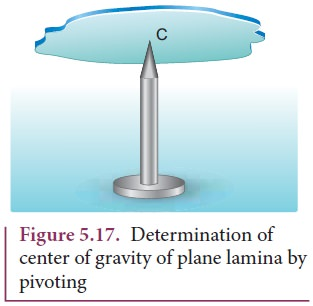Home | | Physics 11th std | Center of Gravity

# Center of Gravity

Each rigid body is made up of several point masses.

Center of Gravity

Each rigid body is made up of several point masses. Such point masses experience gravitational force towards the center of Earth. As the size of Earth is very large compared to any practical rigid body we come across in daily life, these forces appear to be acting parallelly downwards as shown in Figure 5.16.The resultant of these parallel forces always acts through a point. This point is called center of gravity of the body (with respect to Earth). The center of gravity of a body is the point at which the entire weight of the body acts irrespective of the position and orientation of the body. The center of gravity and center of mass of a rigid body coincide when the gravitational field is uniform across the body. The concept of gravitational field is dealt in Unit 6.

We can also determine the center of gravity of a uniform lamina of even an irregular shape by pivoting it at various points by trial and error. The lamina remains horizontal when pivoted at the point where the net gravitational force acts, which is the center of gravity as shown in Figure 5.17. When a body is supported at the center of gravity, the sum of the torques acting on all the point masses of the rigid body becomes zero. Moreover the weight is compensated by the normal reaction force exerted by the pivot. The body is in static equilibrium and hence it remains horizontal.There is also another way to determine the center of gravity of an irregular lamina. If we suspend the lamina from different points like P, Q, R as shown in Figure 5.18, the vertical lines PP', QQ', RR' all pass through the center of gravity.Here, reaction force acting at the point of suspension and the gravitational force acting at the center of gravity cancel each other and the torques caused by them also cancel each other.

Tags : Equilibrium of Rigid Bodies , 11th Physics : UNIT 5 : Motion of System of Particles and Rigid Bodies
Study Material, Lecturing Notes, Assignment, Reference, Wiki description explanation, brief detail
11th Physics : UNIT 5 : Motion of System of Particles and Rigid Bodies : Center of Gravity | Equilibrium of Rigid Bodies